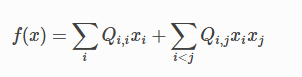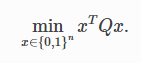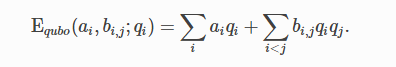# What Is a QUBO?

QUBO stands for quadratic unconstrained binary optimization, and is a problem type traditionally used in computer science. Variables are TRUE and FALSE, states that correspond to 1 and 0 values.

A QUBO problem is defined using an upper-diagonal matrix Q, which is an N x N upper-triangular matrix of real weights, and x, a vector of binary variables, as minimizing the functionwhere the diagonal terms, Q_i,iare the linear coefficients and the nonzero off-diagonal terms are the quadratic coefficients Q_i,j.

This can be expressed more concisely asIn scalar notation, the objective function expressed as a QUBO is as follows:Read more about QUBO problems and how to formulate them for the D-Wave system here.